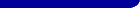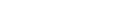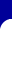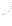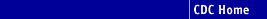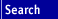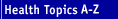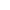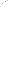Persons using assistive technology might not be able to fully access information in this file. For assistance, please send e-mail to: mmwrq@cdc.gov. Type 508 Accommodation and the title of the report in the subject line of e-mail.

# Appendix

### Traumatic Brain Injury Surveillance: Statistical Methods

Age-adjusted rate estimates were calculated by applying the year 2000 population standard to age-specific crude rate estimates for surveillance year 1997 (Tables 1--5). The year 2000 standard weights (courtesy of the National Center for Health Statistics) are as follows: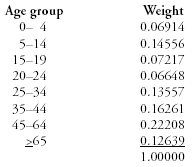Population estimates (by year of age, race, and sex) for 1997 were downloaded for each of the 14 surveillance states from the U.S. Census Bureau website. In any particular analysis, the age-adjusted rate is estimated by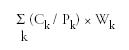where,
Ck denotes the 1997 case count falling into age group k;
Pk denotes the estimated 1997 population for age group k; and
Wk denotes the year 2000 standard weight for age group k.

This formula applies to rate estimates for the entire surveillance population as well as to rate estimates for different subpopulations. The values Ck and Pk always refer to the population of interest; however, the weights Wk are common across all analyses.

#### Stability of Rate Estimates

The stability of each rate estimate (Tables 1--5) is assessed by using the coefficient of variation (C.V.). The C.V. is estimated as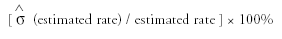Case counts are assumed to follow a Poisson distribution. Under this assumption, the variance of an estimated crude rate is estimated by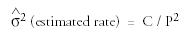where,
C is the case count used to calculate the rate, and
P is the population used to calculate the rate.

The formula for estimating the C.V. of a crude rate simplifies to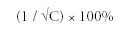For age-adjusted rate estimates, the formula for estimating the variance is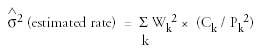where Ck, Pk, and Wk are as defined previously. The square root of this estimated variance is then used when calculating the C.V. as initially defined; no corresponding simplified formula is available.

#### Estimation of Case Distributions

Estimates are provided (Tables 6 and 7) of the distribution of cases across selected one-dimensional classifications. Certain classification variables are core variables, which have a value for every case, whereas other classification variables are extended variables, which have a value only for a sample of cases and involve the use of case weights in the formulation of estimates (see the Sampling Scheme section).

Estimating the distribution of cases across classes associated with a core variable is straightforward because each case implicitly receives a unit weight. The percentage of cases in class k is simply

(Nk / N) × 100%

where,
Nk denotes the number of cases falling into class k, and
N denotes the total number of cases classified.

Estimating the distribution of cases across classes associated with an extended variable is more involved. Each sampled case is assigned a case weight corresponding to the inverse of the sampling fraction for the stratum from which the case was sampled. Because different surveillance states sampled at markedly different rates (and different strata were occasionally sampled at different rates within a state), the case weights cover a wide range. Let

Ws,g denote the case weight assigned to each case sampled from stratum g in state s;
Ns,g denote the total number of sampled cases from stratum g in state s; and
Ns,g,k denote the number of sampled cases from stratum g in state s falling into class k.

Then, the estimated number of cases from stratum g in state s that can be assigned to some class is Ws,g × Ns,g, and the estimated number in class k is Ws,g × Ns,g,k. An estimate of the overall percentage of cases falling into class k, across all states and strata, is given by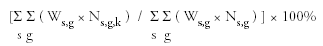The formulas described in this section apply to percentage estimates for the entire surveillance population as well as to percentage estimates for different subpopulations. The values Ns,g and Ns,g,k always refer to the population of interest; however, the case weights Ws,g are common across analyses.

#### Stability of Percentage Estimates

The stability of each reported percentage (Tables 6 and 7) is assessed by using the C.V. For a reported percentage, the C.V. is estimated as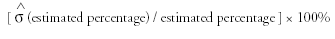The distribution of cases across a set of m > 2 classes is assumed to follow a multinomial distribution; the marginal distribution of cases falling in or out of any particular class is then binomial. Whether the case weights are unit weights (as with core variables) or nonunit weights (as with extended variables), the variance of an estimated percentage PCTk of cases falling into class k can be estimated by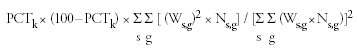where s, g, Ws,g, and Ns,g are as defined previously. Note that when all case weights are unit weights, this formula simplifies to the more familiar form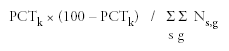### Sampling Scheme for Collecting Data Regarding Extended Variables

For each state designated to collect data for extended variables by means of medical record abstraction, the goal was to obtain information for approximately 1,000 cases. For states with small traumatic brain injury case populations or with comprehensive data collection systems in place (Alaska, Nebraska, Oklahoma, and Rhode Island) this involved sampling at a 100% rate, with abstraction attempted for all hospital discharge cases. Other states (Arizona, Colorado, Louisiana, Minnesota, New York, South Carolina, and Utah) stratified cases (usually according to hospital size, with strata defined as <100 acute care beds or >100 acute care beds) and typically allocated the sample of 1,000 across strata in proportion to stratum size. Multiple states also abstracted data for preadmission death cases, which constituted a separate stratum receiving a proportional share of the sample. Data for preadmission death cases are not analyzed in this report. A simple random sample was then selected within each stratum.

 Use of trade names and commercial sources is for identification only and does not imply endorsement by the U.S. Department of Health and Human Services.References to non-CDC sites on the Internet are provided as a service to MMWR readers and do not constitute or imply endorsement of these organizations or their programs by CDC or the U.S. Department of Health and Human Services. CDC is not responsible for the content of pages found at these sites. URL addresses listed in MMWR were current as of the date of publication.

Disclaimer   All MMWR HTML versions of articles are electronic conversions from ASCII text into HTML. This conversion may have resulted in character translation or format errors in the HTML version. Users should not rely on this HTML document, but are referred to the electronic PDF version and/or the original MMWR paper copy for the official text, figures, and tables. An original paper copy of this issue can be obtained from the Superintendent of Documents, U.S. Government Printing Office (GPO), Washington, DC 20402-9371; telephone: (202) 512-1800. Contact GPO for current prices.

**Questions or messages regarding errors in formatting should be addressed to mmwrq@cdc.gov.

Page converted: 6/11/2003Morbidity and Mortality Weekly Report Centers for Disease Control and Prevention 1600 Clifton Rd, MailStop E-90, Atlanta, GA 30333, U.S.A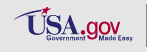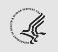Department of Healthand Human Services